# How do gases react to temperature

##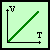Gay Lussac's Gas Act

Page - 7 -

II. The gas laws

Joseph Gay-Lussac investigated the change in volume of a gas at different temperatures. He always kept the pressure the same (= constant) in these experiments. He did this by trapping the gas and heating or cooling it. The first publications about it appeared between 1802 and 1808.
In his experiments he used various gases, such as oxygen (O2), Hydrogen (H2) or nitrogen (N2). As a result, he found that the volume of these gases has the same dependence on temperature. If the gas is heated by a certain factor, the volume of the gas is also increased by a certain factor. On the other hand, as it cooled, the volume became smaller.Derivation of the formula:
The temperature is proportional to the volume. This means that if you, for example, increase the temperature by twice, then the volume of the gas also doubles.
The volume is denoted by V and the temperature by Q.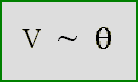(1)

If you represent the whole thing graphically and plot the volume against the temperature, you get the following picture: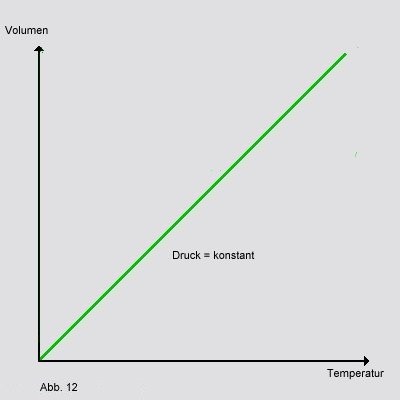Another quantity is also introduced, namely V0. This is the volume of the gas at a temperature of 0 C. Gay-Lussac now set up a formula so that he could also calculate with the connection he had found: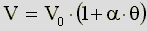(2)

In this formula, the symbol a is the so-called. thermal expansion coefficient. You need this number so that you can write an equation, because you are working with a proportionality relationship (see formula (1)) can not count. Through measurements, Gay-Lussac found a value of 1/267 for a. However, this value was not exactly correct, as Regnault found out in 1847 with a better experimental set-up. The exact value is 1 / 273.15.
If you plug this value into the equation, it looks like this: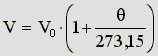(3)

From this equation we can now see that a gas expands by 1 / 273.15 when it is heated from 0 C to 1 C (but only at constant pressure!).
At 273.15 it is exactly the value at which the absolute zero point is in the negative Celsius range. Instead of this number you can also use T0 write. In addition, a new scale is defined, which is measured in Kelvin instead of degrees Celsius:The temperature that can now be read off the scale is called the absolute temperature T and is given in Kelvin. From Fig. 12 you can see that e.g. 27 C corresponds to an absolute temperature of 300K.
There is the following relationship between the different temperatures (in C and K):(4)

Since T0 has a fixed value, namely 273, this formula can always be used to convert degrees Celsius to Kelvin and vice versa.
Solving this equation for Q, the following happens: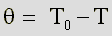(5)

This is now put into a formula (3) and thus obtains this equation:(6)

If you simplify this further (by multiplying and adding), you get that Gay-Lussac gas law, which describes the relationship between temperature and volume of a gas at constant pressure: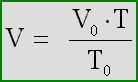(7)

However, this relationship only relates to the reference values ​​for volume and temperature. But one can also apply this law more generally, and instead of T.0 and V0 Use other starting values ​​for volume and temperature.

Example:
If you put one of the alkali metals (see periodic table, first column) in a bowl with water, it reacts violently. It floats around on the surface and dissolves with evolution of gas. The resulting gas is hydrogen (H.2).Given: At a temperature of 15 C, the height is 370 cm3 Hydrogen formed.

Question: What is the volume of hydrogen at a temperature of 25 C?

Solution: Since the pressure does not change, the gas law of Gay Lussac apply:The given values ​​are now inserted into this equation, with the temperature values ​​as from the equations (5) to (7) must be converted from C to the absolute temperature scale (Kelvin), and the solution is obtained directly from this:Answer: The volume of the hydrogen is about 383 cm3 at 25 C.